# 3 Linear waves

As a first step, we linearize Eqs. (9)-(12), to study small-amplitude harmonic waves of frequency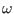and wavenumber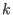. The linear dispersion relation for electron-acoustic waves then reads: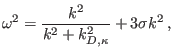(14)

where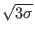is essentially the (normalized) cool electron thermal velocity. After taking account of differences in normalization, this agrees with the form found in .

We note the appearance of a normalized-dependent screening factor (scaled Debye wavenumber)in the denominator, defined by(15)

Since this is the inverse of the (Debye) screening length, we notice that the latter in fact decreases due to an excess in suprathermal electrons (i.e.,for any finite value of). This is in agreement with Refs. [40,41,42]; note also the discussion in Ref. .

From Eq. (14), we see that the frequency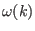, and hence also the phase speed, increases with higher temperature ratio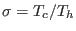. However, this is usually a small correction to the dominant first term on the righthand side of (15). For large wavelength values (small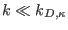), the phase speed is given by(16)

while on the other hand, the thermal contribution is dominant for high wavenumber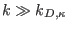, i.e.(17)

However, we should recall from kinetic theory[1,2,3,5] that both for very long wavelengths () and very short wavelengths (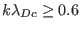), the wave is strongly damped, and thus these limits may be of academic interest only. The mode is weakly damped only for intermediate wavelength values, where its acoustic nature is not manifest. [1,2,3,5,29] Here,is the cool electron Debye length.Restoring dimensions for a moment, the dispersion relation becomes(18)

where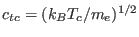is the cool electron thermal speed andis the hot electron Debye length defined by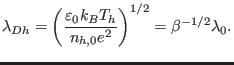(19)

It appears appropriate to compare the above results with earlier results, in the linear regime. First of all, we note that Ref.  has adopted a kinetic description of electron-acoustic waves in suprathermal plasmas. For this purpose, Eq. (18) may be cast in the form,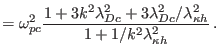(20)

Here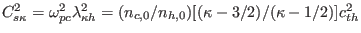is the electron acoustic speed in a suprathermal plasma, while the effective shielding length is given by. Our Eq. (20) above agrees with relation (3) in Ref.  (upon considering the limittherein). In the limit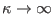(Maxwellian distribution), Eq. (20) recovers precisely Eq. (1) in Ref. , upon considering the limit. Furthermore, one recovers exactly Eq. (5) in Ref.  for Maxwellian plasma in the cold-electron limit ().

In Figure 1, we depict the dispersion curve of the electron-acoustic mode, showing the effect of varying the values of the spectral indexand the density ratio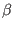. It is confirmed numerically that the phase speed (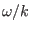) increases weakly with a reduction in suprathermal particle excess, as the Maxwellian is approached, and that there is a significant reduction in phase speed as the plasma model changes from one in which the cool electrons dominate, to one which is dominated by the hot electron density.

Ashkbiz Danehkar
2018-03-28